# 2020更新，Vue仿探探拖拽卡片的效果

35,897

`绝对原创，如有雷同，纯属对方抄袭`😁

# 先叠起来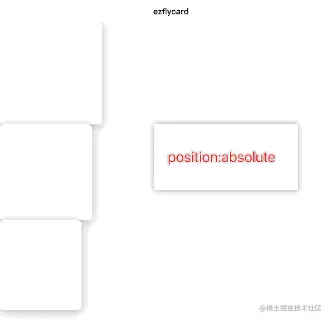# 拖动第一张卡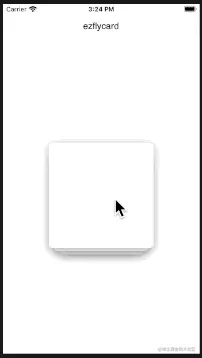``````touchStart:function(e){
var curTouch=e.touches;
this.startLeft=curTouch.clientX-this.left;
this.startTop=curTouch.clientY-this.top;
}
touchMove:function(e){
var curTouch=e.touches;
this.left=curTouch.clientX-this.startLeft;
this.top=curTouch.clientY-this.startTop;
}
``````

# 飞出去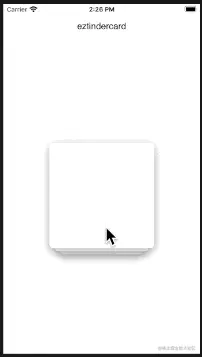``````var angle=Math.atan2((当前坐标.y-起点坐标.y), (当前坐标.x-起点坐标.x));
``````

``````this.left=Math.cos(angle)*this.throwDistance;
``````

``````this.top=Math.sin(angle)*this.throwDistance;
``````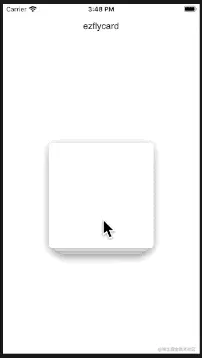``````//计算两点之间的直线距离
getDistance:function(x1, y1, x2, y2) {
var _x = Math.abs(x1 - x2);
var _y = Math.abs(y1 - y2);
return Math.sqrt(_x * _x + _y * _y);
}

var distance=this.getDistance(0,0,this.left,this.top);
if(distance>this.throwTriggerDistance){
this.makeCardThrow();
}else{
this.makeCardBack();
}
``````

# 下层的卡片上推

• 第2张卡片变更为原本第1张卡片的位置和大小
``````this.width2=this.cardWidth;
this.height2=this.cardHeight;
this.left2=0;
this.top2=0;
``````
• 第3张卡片变更为原本第2张卡片的位置和大小
``````this.width3=(this.cardWidth-this.leftPad*2);
``````
• 第4张卡片原本是透明的，现在变为第3张卡片的位置和大小
``````this.width4=(this.cardWidth-this.leftPad*4);
this.opacity4=1;
``````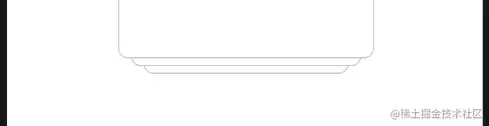# 重置所有卡片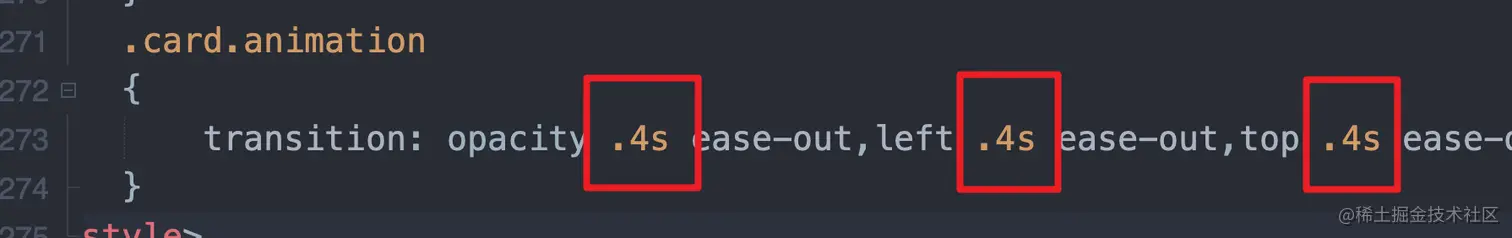``````this.onThrowStart();
setTimeout(function(){
that.isThrow=false;
that.isAnimating=false;
that.onThrowDone();
that.resetAllCard();
},400);
``````

# 组件化

``````//提供几个事件，分别是拖动时，拖动结束，飞卡结束，飞卡失败（回位）
@onDragMove='onCardDragMove'
@onDragStop='onCardDragStop'
@onThrowDone='onCardThrowDone'
@onThrowFail='onCardThrowFail'

//参数就不细说了，都能看明白
:cardWidth="200"
:cardHeight="200"
cardBgColor="#fff"
:throwTriggerDistance="100"
dragDirection="all"
:hasBorder="true"
``````

``````//firstCard，secondCard，thirdCard
<slot name="firstCard"></slot>
``````

# 现在来模仿几个效果

## 某乎的推荐回答

``````@onDragMove='onCardDragMove'
@onDragStop='onCardDragStop'
@onThrowDone='onCardThrowDone'
:cardWidth="300"
:cardHeight="120"
:throwTriggerDistance="100"
dragDirection="horizontal"
``````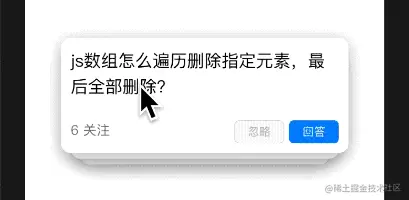## 探探的效果

``````onCardDragMove(obj){
if(obj.left<-10){
this.actionName="不喜欢";
}else if(obj.left>10){
this.actionName="喜欢";
}else{
this.actionName="";
}
}
``````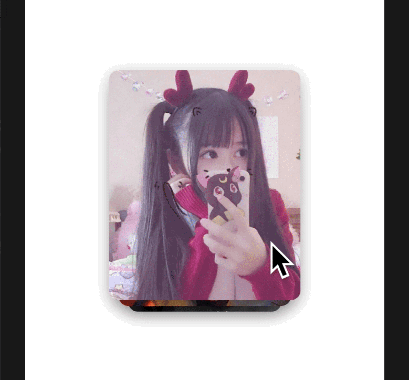# 源码仓库

github.com/ezshine/ezf…